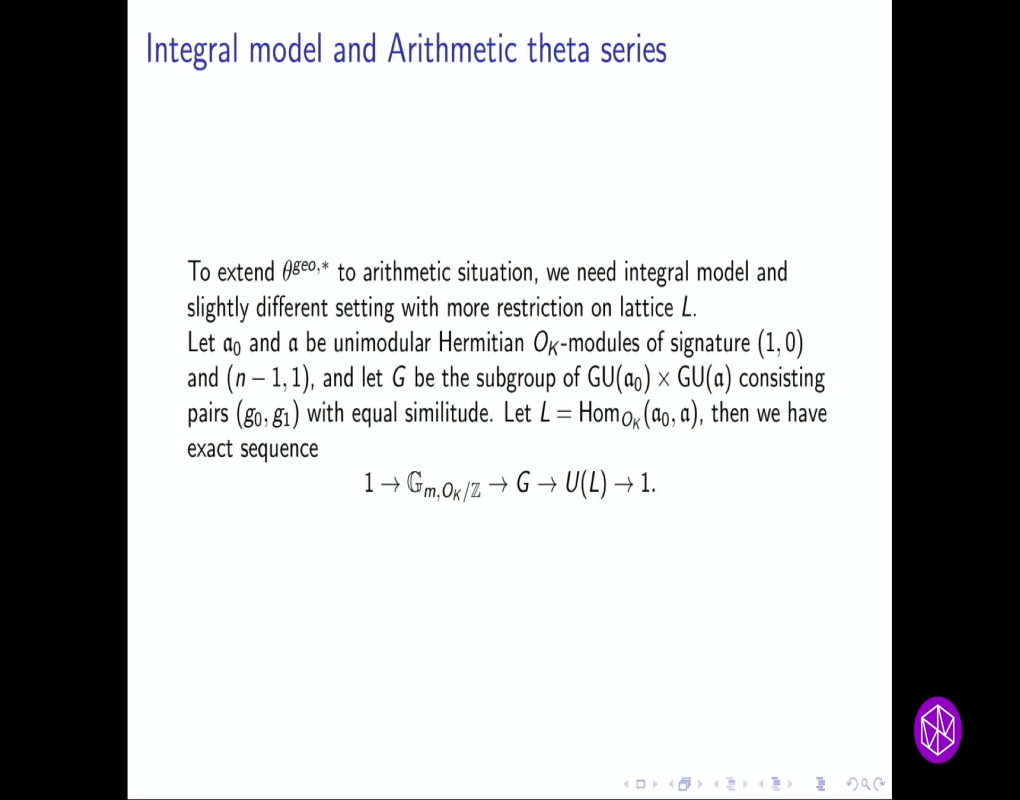### Videos

#### Algebraic Cycles, L-Values, and Euler Systems "Shimura Varieties and L-Functions" Topical Workshop: Arithmetic Theta Kernel and Liftings

##### Presenter
March 13, 2023
Keywords:
• Kudla program
• arithmetic Siegel-Weil formulas
• modularity
MSC:
• 11G18
• 11G40
• 11F87##### Abstract
Let $K=\Q(\sqrt d)$ be an imaginary quadratic field. Let $L$ be a unimodular $O_K$-lattice of signature $(n-1, 1)$, and let $\mathcal X$ be the compactified Shimura variety over $O_K$ associated at $L$. In this talk, we describe roughly how to construct an arithmetic theta series (arithmetic theta kernel), and prove that it is a modular form of level $d$, weight $n$, character $\chi_d^n$ with values in $\widehat{CH}^1(\mathcal X^*)$. Using this arithmetic theta kernel, we can produce classical modular forms from arithmetic $1$-cycles (Faltings’ height pairing) and produce arithmetic divisors from classical cusp forms. This is joint work with Bruinier, Howard, Kudla, and Rapoport for $n \ge 3$. The case $2$ is a joint work in progress with Qiao He and Yousheng Shi.